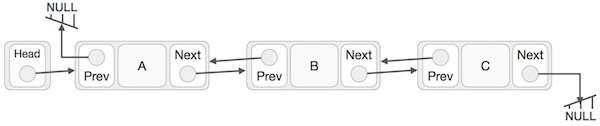# Program to find size of Doubly Linked List in C++

In this problem, we are given a doubly linked list. Our task is to create a program to find size of Doubly Linked List in C++.

Doubly Linked List is a special type of Linked list in which navigation is possible in both ways, either forward and backward easily as compared to Single Linked List. The following are the important terms to understand the concept of doubly linked lists.

Representation of a Doubly Linked List −Problem Description − We will be given a doubly linked list of the above type. And we will find the size (length) of it.

Let’s take an example to understand the problem,

## Input

the above linked list A <-> B <-> C.

## Output

3

## Solution Approach

To find the size of the doubly linked list, we need to traverse the doubly linked list and keep track of the length with the length valable.

### Algorithm

Initialise − length = 0, *temp = head

• Step 1 − Traverse the list i.e. do till the temp != NULL.
• Step 1.1 − Increase length, length++
• Step 1.2 − Update pointer, temp = temp -> next.
• Step 2 − print length.

Program to illustrate the working of our solution

## Example

Live Demo

#include <iostream>
using namespace std;
struct doublyLL {
char val;
struct doublyLL *next;
struct doublyLL *prev;
};
void insertNode(struct doublyLL** head_ref, int value){
struct doublyLL* new_node = new doublyLL;
new_node->val = value;
new_node->prev = NULL;
}
int calcDLLSize(struct doublyLL *temp) {
int length = 0;
while (temp != NULL){
temp = temp->next;
length++;
}
return length;
}
int main(){
}
The size of Doubly Linked List is 6